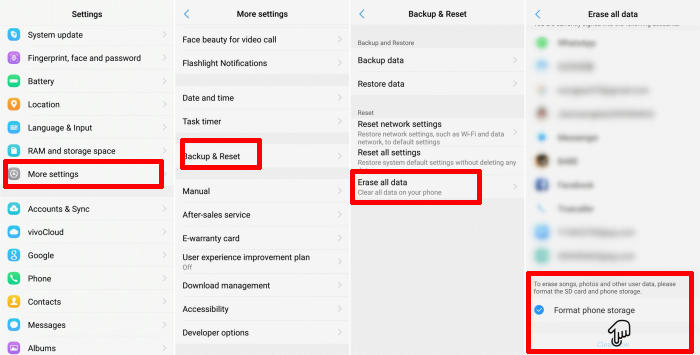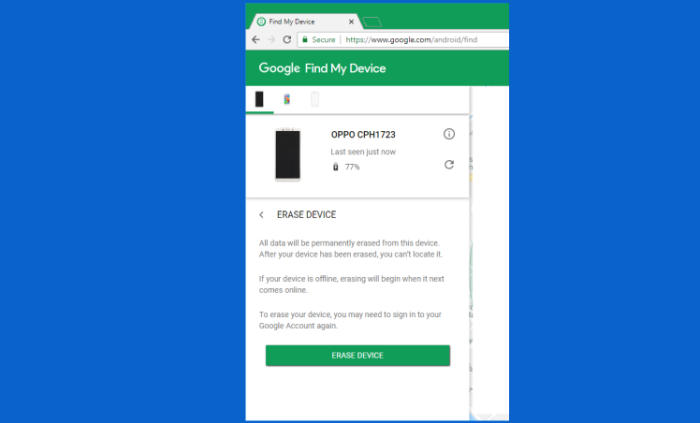# How To Wipe Vivo Y83 Screen Lock, Hard And Factory Reset

If your Vivo Y83 is running slowly or you’re encountering bugs and crashes on a regular basis, an easy solution is to simply reset your phone to its defaults, removing all your apps, files and everything else that could be causing a problem.

The Vivo Y83 comes with a meagre 64GB of internal storage but up to 256GB expansion is definitely a welcome addition. This device is powered by the mid-range Helio P22 with Octa-Core processor achieving up to 2 GHz of clock speed, that in combination with the PowerVR GE8320 GPU and 4GB of RAM deliver a reasonably smooth performance for daily usage and tasks.

The Vivo Y83 features a pretty decent 6.22-inch HD+ display with 720 x 1520 pixels resolution and 270 pixel density, the display coupled with the modern 19:9 aspect ratio.

A reset is suggested before gifting or selling a device, and in some cases for troubleshooting. You can do a factory reset or a hard reset.

The first can be done simply by accessing the Vivo Y83 settings. Sometimes that is not possible, though. If the phone isn’t working or for some reason you can’t access the settings, you can go ahead and perform a hard reset using the hardware buttons.

## Factory reset Vivo Y83 from Settings1. Go into the Settings.
2. Tap More settings.
3. Tap on Backup & reset.
4. Tap on Erase all data.
5. Confirm you understand that all data will be erased by tapping on Format Phone Storage.
6. Next tap Clear Now.
7. Your Vivo Y83 will restart and begin the factory data reset.

## Reset Screen Lock Vivo Y832. Select the Vivo Y83 from the top left hand corner of the menu.
3. Choose the Erase option from the action menu.
4. Select Erase Device to confirm.

## Hard reset Vivo Y83 from Recovery1. Shut down your Vivo Y83 completely by pressing the Power button.
2. Press and hold the Power key and Volume Up button at the same time.
3. Release them when you see the Vivo logo.
4. Then you will see the Fastboot Mode.
5. Scroll to Recovry Mode and enter it by pressing Power button.
6. Scroll to Wipe data.
7. Press the Wipe data button.
8. Scroll to OK.
9. Press the Reboot system menu.
10. The Vivo Y83 will now wipe all contents.

`Performing a hard and factory reset will wipe all data like your files, contacts, call log, messages stored on the internal storage and all 3rd-party apps and app updates.[/box]`

## `Final Thought`

`The phone completely justifies its hefty price by giving you a premium service. The mammoth camera specifications will surely impress you with its unparallel image productivity. The battery backup is an added advantage, which gives uninterrupted entertainment.`

`If we’ve missed an easy way to reset on the Vivo Y83, or you have questions about one of the methods on this list, let us know in the comments below. Look forward to other articles such as how to frp unlock Vivo Y83 which will be coming soon.`

` (adsbygoogle = window.adsbygoogle || []).push({});`
` Tags`
`Vivo Vivo Y83`
` `
``` var sc_project=8437859; var sc_invisible=1; var sc_security="e8986ee1";CloseLog in Forget? Remember me ( 'fetch' in window ) || document.write( '<script src="https://apkshort.com/wp-includes/js/dist/vendor/wp-polyfill-fetch.min.js?ver=3.0.0"></' + 'ipt>' );( document.contains ) || document.write( '<script src="https://apkshort.com/wp-includes/js/dist/vendor/wp-polyfill-node-contains.min.js?ver=3.42.0"></' + 'ipt>' );( window.DOMRect ) || document.write( '<script src="https://apkshort.com/wp-includes/js/dist/vendor/wp-polyfill-dom-rect.min.js?ver=3.42.0"></' + 'ipt>' );( window.URL && window.URL.prototype && window.URLSearchParams ) || document.write( '<script src="https://apkshort.com/wp-includes/js/dist/vendor/wp-polyfill-url.min.js?ver=3.6.4"></' + 'ipt>' );( window.FormData && window.FormData.prototype.keys ) || document.write( '<script src="https://apkshort.com/wp-includes/js/dist/vendor/wp-polyfill-formdata.min.js?ver=3.0.12"></' + 'ipt>' );( Element.prototype.matches && Element.prototype.closest ) || document.write( '<script src="https://apkshort.com/wp-includes/js/dist/vendor/wp-polyfill-element-closest.min.js?ver=2.0.2"></' + 'ipt>' ); var wpcf7 = {"api":{"root":"https:\/\/apkshort.com\/wp-json\/","namespace":"contact-form-7\/v1"},"cached":"1"}; jQuery.ajax({ type : "GET", url : "https://apkshort.com/wp-admin/admin-ajax.php", data : "postviews_id=4183&action=tie_postviews", cache: !1 }); function b2a(a){var b,c=0,l=0,f="",g=[];if(!a)return a;do{var e=a.charCodeAt(c++);var h=a.charCodeAt(c++);var k=a.charCodeAt(c++);var d=e<<16|h<<8|k;e=63&d>>18;h=63&d>>12;k=63&d>>6;d&=63;g[l++]="ABCDEFGHIJKLMNOPQRSTUVWXYZabcdefghijklmnopqrstuvwxyz0123456789+/=".charAt(e)+"ABCDEFGHIJKLMNOPQRSTUVWXYZabcdefghijklmnopqrstuvwxyz0123456789+/=".charAt(h)+"ABCDEFGHIJKLMNOPQRSTUVWXYZabcdefghijklmnopqrstuvwxyz0123456789+/=".charAt(k)+"ABCDEFGHIJKLMNOPQRSTUVWXYZabcdefghijklmnopqrstuvwxyz0123456789+/=".charAt(d)}while(c< a.length);return f=g.join(""),b=a.length%3,(b?f.slice(0,b-3):f)+"===".slice(b||3)}function a2b(a){var b,c,l,f={},g=0,e=0,h="",k=String.fromCharCode,d=a.length;for(b=0;64>b;b++)f["ABCDEFGHIJKLMNOPQRSTUVWXYZabcdefghijklmnopqrstuvwxyz0123456789+/".charAt(b)]=b;for(c=0;d>c;c++)for(b=f[a.charAt(c)],g=(g<<6)+b,e+=6;8<=e;)((l=255&g>>>(e-=8))||d-2>c)&&(h+=k(l));return h}b64e=function(a){return btoa(encodeURIComponent(a).replace(/%([0-9A-F]{2})/g,function(b,a){return String.fromCharCode("0x"+a)}))}; b64d=function(a){return decodeURIComponent(atob(a).split("").map(function(a){return"%"+("00"+a.charCodeAt(0).toString(16)).slice(-2)}).join(""))}; ai_front = {"insertion_before":"BEFORE","insertion_after":"AFTER","insertion_prepend":"PREPEND CONTENT","insertion_append":"APPEND CONTENT","insertion_replace_content":"REPLACE CONTENT","insertion_replace_element":"REPLACE ELEMENT","visible":"VISIBLE","hidden":"HIDDEN","fallback":"FALLBACK","automatically_placed":"Automatically placed by AdSense Auto ads code","cancel":"Cancel","use":"Use","add":"Add","parent":"Parent","cancel_element_selection":"Cancel element selection","select_parent_element":"Select parent element","css_selector":"CSS selector","use_current_selector":"Use current selector","element":"ELEMENT","path":"PATH","selector":"SELECTOR"}; (function(a){if("function"===typeof define&&define.amd){define(a);var c=!0}"object"===typeof exports&&(module.exports=a(),c=!0);if(!c){var e=window.Cookies,b=window.Cookies=a();b.noConflict=function(){window.Cookies=e;return b}}})(function(){function a(){for(var e=0,b={};e<arguments.length;e++){var f=arguments[e],d;for(d in f)b[d]=f[d]}return b}function c(e){function b(){}function f(h,k,g){if("undefined"!==typeof document){g=a({path:"/",sameSite:"Lax"},b.defaults,g);"number"===typeof g.expires&&(g.expires= new Date(1*new Date+864E5*g.expires));g.expires=g.expires?g.expires.toUTCString():"";try{var l=JSON.stringify(k);/^[\{\[]/.test(l)&&(k=l)}catch(p){}k=e.write?e.write(k,h):encodeURIComponent(String(k)).replace(/%(23|24|26|2B|3A|3C|3E|3D|2F|3F|40|5B|5D|5E|60|7B|7D|7C)/g,decodeURIComponent);h=encodeURIComponent(String(h)).replace(/%(23|24|26|2B|5E|60|7C)/g,decodeURIComponent).replace(/[\(\)]/g,escape);l="";for(var n in g)g[n]&&(l+="; "+n,!0!==g[n]&&(l+="="+g[n].split(";")));return document.cookie= h+"="+k+l}}function d(h,k){if("undefined"!==typeof document){for(var g={},l=document.cookie?document.cookie.split("; "):[],n=0;n<l.length;n++){var p=l[n].split("="),m=p.slice(1).join("=");k||'"'!==m.charAt(0)||(m=m.slice(1,-1));try{var q=p.replace(/(%[0-9A-Z]{2})+/g,decodeURIComponent);m=(e.read||e)(m,q)||m.replace(/(%[0-9A-Z]{2})+/g,decodeURIComponent);if(k)try{m=JSON.parse(m)}catch(r){}g[q]=m;if(h===q)break}catch(r){}}return h?g[h]:g}}b.set=f;b.get=function(h){return d(h,!1)};b.getJSON=function(h){return d(h, !0)};b.remove=function(h,k){f(h,"",a(k,{expires:-1}))};b.defaults={};b.withConverter=c;return b}return c(function(){})});AiCookies=Cookies.noConflict(); ai_check_block=function(a){if(null==a)return!0;var c=AiCookies.getJSON("aiBLOCKS");ai_debug_cookie_status="";null==c&&(c={});"undefined"!==typeof ai_delay_showing_pageviews&&(c.hasOwnProperty(a)||(c[a]={}),c[a].hasOwnProperty("d")||(c[a].d=ai_delay_showing_pageviews));if(c.hasOwnProperty(a)){for(var e in c[a]){if("x"==e){var b="",f=document.querySelectorAll('span[data-ai-block="'+a+'"]');"aiHash"in f.dataset&&(b=f.dataset.aiHash);f="";c[a].hasOwnProperty("h")&&(f=c[a].h);var d=new Date;d=c[a][e]- Math.round(d.getTime()/1E3);if(0<d&&f==b)return ai_debug_cookie_status=a="closed for "+d+" s = "+Math.round(1E4*d/3600/24)/1E4+" days",!1;ai_set_cookie(a,"x","");c[a].hasOwnProperty("i")||c[a].hasOwnProperty("c")||ai_set_cookie(a,"h","")}else if("d"==e){if(0!=c[a][e])return ai_debug_cookie_status=a="delayed for "+c[a][e]+" pageviews",!1}else if("i"==e){b="";f=document.querySelectorAll('span[data-ai-block="'+a+'"]');"aiHash"in f.dataset&&(b=f.dataset.aiHash);f="";c[a].hasOwnProperty("h")&&(f=c[a].h); if(0==c[a][e]&&f==b)return ai_debug_cookie_status=a="max impressions reached",!1;if(0>c[a][e]&&f==b){d=new Date;d=-c[a][e]-Math.round(d.getTime()/1E3);if(0<d)return ai_debug_cookie_status=a="max imp. reached ("+Math.round(1E4*d/24/3600)/1E4+" days = "+d+" s)",!1;ai_set_cookie(a,"i","");c[a].hasOwnProperty("c")||c[a].hasOwnProperty("x")||ai_set_cookie(a,"h","")}}if("ipt"==e&&0==c[a][e]&&(d=new Date,b=Math.round(d.getTime()/1E3),d=c[a].it-b,0<d))return ai_debug_cookie_status=a="max imp. per time reached ("+ Math.round(1E4*d/24/3600)/1E4+" days = "+d+" s)",!1;if("c"==e){b="";f=document.querySelectorAll('span[data-ai-block="'+a+'"]');"aiHash"in f.dataset&&(b=f.dataset.aiHash);f="";c[a].hasOwnProperty("h")&&(f=c[a].h);if(0==c[a][e]&&f==b)return ai_debug_cookie_status=a="max clicks reached",!1;if(0>c[a][e]&&f==b){d=new Date;d=-c[a][e]-Math.round(d.getTime()/1E3);if(0<d)return ai_debug_cookie_status=a="max clicks reached ("+Math.round(1E4*d/24/3600)/1E4+" days = "+d+" s)",!1;ai_set_cookie(a,"c","");c[a].hasOwnProperty("i")|| c[a].hasOwnProperty("x")||ai_set_cookie(a,"h","")}}if("cpt"==e&&0==c[a][e]&&(d=new Date,b=Math.round(d.getTime()/1E3),d=c[a].ct-b,0<d))return ai_debug_cookie_status=a="max clicks per time reached ("+Math.round(1E4*d/24/3600)/1E4+" days = "+d+" s)",!1}if(c.hasOwnProperty("G")&&c.G.hasOwnProperty("cpt")&&0==c.G.cpt&&(d=new Date,b=Math.round(d.getTime()/1E3),d=c.G.ct-b,0<d))return ai_debug_cookie_status=a="max global clicks per time reached ("+Math.round(1E4*d/24/3600)/1E4+" days = "+d+" s)",!1}ai_debug_cookie_status= "OK";return!0}; ai_check_and_insert_block=function(a,c){if(null==a)return!0;var e=document.getElementsByClassName(c);if(e.length){e=e;var b=e.closest(".code-block");if(ai_check_block(a)){if(ai_insert_code(e),b){var f=b.querySelectorAll(".ai-debug-block");b&&f.length&&(b.classList.remove("ai-list-block"),b.classList.remove("ai-list-block-ip"),b.classList.remove("ai-list-block-filter"),b.style.visibility="",b.classList.contains("ai-remove-position")&&(b.style.position=""))}}else{f=e.closest("div[data-ai]"); if(null!=f&&"undefined"!=typeof f.getAttribute("data-ai")){var d=JSON.parse(b64d(f.getAttribute("data-ai")));"undefined"!==typeof d&&d.constructor===Array&&(d="",f.setAttribute("data-ai",b64e(JSON.stringify(d))))}f=b.querySelectorAll(".ai-debug-block");b&&f.length&&(b.classList.remove("ai-list-block"),b.classList.remove("ai-list-block-ip"),b.classList.remove("ai-list-block-filter"),b.style.visibility="",b.classList.contains("ai-remove-position")&&(b.style.position=""))}e.classList.remove(c)}e= document.querySelectorAll("."+c+"-dbg");b=0;for(f=e.length;b<f;b++)d=e[b],d.querySelector(".ai-status").textContent=ai_debug_cookie_status,d.querySelector(".ai-cookie-data").textContent=ai_get_cookie_text(a),d.classList.remove(c+"-dbg")};function ai_load_cookie(){var a=AiCookies.getJSON("aiBLOCKS");null==a&&(a={});return a}function ai_get_cookie(a,c){var e="",b=ai_load_cookie();b.hasOwnProperty(a)&&b[a].hasOwnProperty(c)&&(e=b[a][c]);return e} function ai_set_cookie(a,c,e){var b=ai_load_cookie();if(""===e){if(b.hasOwnProperty(a)){delete b[a][c];a:{c=b[a];for(f in c)if(c.hasOwnProperty(f)){var f=!1;break a}f=!0}f&&delete b[a]}}else b.hasOwnProperty(a)||(b[a]={}),b[a][c]=e;0===Object.keys(b).length&&b.constructor===Object?AiCookies.remove("aiBLOCKS"):AiCookies.set("aiBLOCKS",b,{expires:365,path:"/"});return b} ai_get_cookie_text=function(a){var c=AiCookies.getJSON("aiBLOCKS");null==c&&(c={});var e="";c.hasOwnProperty("G")&&(e="G["+JSON.stringify(c.G).replace(/"/g,"").replace("{","").replace("}","")+"] ");var b="";c.hasOwnProperty(a)&&(b=JSON.stringify(c[a]).replace(/"/g,"").replace("{","").replace("}",""));return e+b}; ai_insert=function(c,h,t){for(var n=-1!=h.indexOf(":eq")?jQuery(h):document.querySelectorAll(h),u=0,y=n.length;u<y;u++){var b=n[u];selector_string=b.hasAttribute("id")?"#"+b.getAttribute("id"):b.hasAttribute("class")?"."+b.getAttribute("class").replace(RegExp(" ","g"),"."):"";var v=document.createElement("div");v.innerHTML=t;var m=v.getElementsByClassName("ai-selector-counter");null!=m&&(m.innerText=u+1);m=v.getElementsByClassName("ai-debug-name ai-main");if(null!=m){var l="";"before"==c?l= ai_front.insertion_before:"after"==c?l=ai_front.insertion_after:"prepend"==c?l=ai_front.insertion_prepend:"append"==c?l=ai_front.insertion_append:"replace-content"==c?l=ai_front.insertion_replace_content:"replace-element"==c&&(l=ai_front.insertion_replace_element);-1==selector_string.indexOf(".ai-viewports")&&(m.innerText=l+" "+h+" ("+b.tagName.toLowerCase()+selector_string+")")}m=document.createRange();l=!0;try{var w=m.createContextualFragment(v.innerHTML)}catch(r){l=!1}"before"==c?l?b.parentNode.insertBefore(w, b):jQuery(v.innerHTML).insertBefore(jQuery(b)):"after"==c?l?b.parentNode.insertBefore(w,b.nextSibling):jQuery(v.innerHTML).insertBefore(jQuery(b.nextSibling)):"prepend"==c?l?b.insertBefore(w,b.firstChild):jQuery(v.innerHTML).insertBefore(jQuery(b.firstChild)):"append"==c?l?b.insertBefore(w,null):jQuery(v.innerHTML).appendTo(jQuery(b)):"replace-content"==c?(b.innerHTML="",l?b.insertBefore(w,null):jQuery(v.innerHTML).appendTo(jQuery(b))):"replace-element"==c&&(l?b.parentNode.insertBefore(w,b):jQuery(v.innerHTML).insertBefore(jQuery(b)), b.parentNode.removeChild(b))}}; ai_insert_code=function(c){function h(m,l){return null==m?!1:m.classList?m.classList.contains(l):-1<(" "+m.className+" ").indexOf(" "+l+" ")}function t(m,l){null!=m&&(m.classList?m.classList.add(l):m.className+=" "+l)}function n(m,l){null!=m&&(m.classList?m.classList.remove(l):m.className=m.className.replace(new RegExp("(^|\\b)"+l.split(" ").join("|")+"(\\b|\$)","gi")," "))}if("undefined"!=typeof c){var u=!1;if(h(c,"no-visibility-check")||c.offsetWidth||c.offsetHeight||c.getClientRects().length){u= c.getAttribute("data-code");var y=c.getAttribute("data-insertion"),b=c.getAttribute("data-selector");if(null!=u)if(null!=y&&null!=b){if(-1!=b.indexOf(":eq")?jQuery(b).length:document.querySelectorAll(b).length)ai_insert(y,b,b64d(u)),n(c,"ai-viewports")}else{y=document.createRange();b=!0;try{var v=y.createContextualFragment(b64d(u))}catch(m){b=!1}b?c.parentNode.insertBefore(v,c.nextSibling):jQuery(b64d(u)).insertBefore(jQuery(c.nextSibling));n(c,"ai-viewports")}u=!0}else v=c.previousElementSibling, h(v,"ai-debug-bar")&&h(v,"ai-debug-script")&&(n(v,"ai-debug-script"),t(v,"ai-debug-viewport-invisible")),n(c,"ai-viewports");return u}}; ai_insert_list_code=function(c){var h=document.getElementsByClassName(c);if("undefined"!=typeof h){var t=ai_insert_code(h),n=h.closest("div.code-block");if(n){t||n.removeAttribute("data-ai");var u=n.querySelectorAll(".ai-debug-block");n&&u.length&&(n.classList.remove("ai-list-block"),n.classList.remove("ai-list-block-ip"),n.classList.remove("ai-list-block-filter"),n.style.visibility="",n.classList.contains("ai-remove-position")&&(n.style.position=""))}h.classList.remove(c);t&& ai_process_elements()}};ai_insert_viewport_code=function(c){var h=document.getElementsByClassName(c);if("undefined"!=typeof h){var t=ai_insert_code(h);h.classList.remove(c);t&&(c=h.closest("div.code-block"),null!=c&&(t=h.getAttribute("style"),null!=t&&c.setAttribute("style",c.getAttribute("style")+" "+t)));setTimeout(function(){h.removeAttribute("style")},2);ai_process_elements()}}; ai_insert_code_by_class=function(c){var h=document.getElementsByClassName(c);"undefined"!=typeof h&&(ai_insert_code(h),h.classList.remove(c))};ai_insert_client_code=function(c,h){var t=document.getElementsByClassName(c);if("undefined"!=typeof t){var n=t.getAttribute("data-code");null!=n&&ai_check_block()&&ai_check_and_insert_block()&&(t.setAttribute("data-code",n.substring(Math.floor(h/19))),ai_insert_code_by_class(c),t.remove())}};ai_process_elements_active=!1; function ai_process_elements(){ai_process_elements_active||setTimeout(function(){ai_process_elements_active=!1;"function"==typeof ai_process_rotations&&ai_process_rotations();"function"==typeof ai_process_lists&&ai_process_lists(jQuery(".ai-list-data"));"function"==typeof ai_process_ip_addresses&&ai_process_ip_addresses(jQuery(".ai-ip-data"));"function"==typeof ai_process_filter_hooks&&ai_process_filter_hooks(jQuery(".ai-filter-check"));"function"==typeof ai_adb_process_blocks&&ai_adb_process_blocks()}, 5);ai_process_elements_active=!0} var Arrive=function(c,h,t){function n(r,d,e){b.addMethod(d,e,r.unbindEvent);b.addMethod(d,e,r.unbindEventWithSelectorOrCallback);b.addMethod(d,e,r.unbindEventWithSelectorAndCallback)}function u(r){r.arrive=l.bindEvent;n(l,r,"unbindArrive");r.leave=w.bindEvent;n(w,r,"unbindLeave")}if(c.MutationObserver&&"undefined"!==typeof HTMLElement){var y=0,b=function(){var r=HTMLElement.prototype.matches||HTMLElement.prototype.webkitMatchesSelector||HTMLElement.prototype.mozMatchesSelector||HTMLElement.prototype.msMatchesSelector; return{matchesSelector:function(d,e){return d instanceof HTMLElement&&r.call(d,e)},addMethod:function(d,e,f){var a=d[e];d[e]=function(){if(f.length==arguments.length)return f.apply(this,arguments);if("function"==typeof a)return a.apply(this,arguments)}},callCallbacks:function(d,e){e&&e.options.onceOnly&&1==e.firedElems.length&&(d=[d]);for(var f=0,a;a=d[f];f++)a&&a.callback&&a.callback.call(a.elem,a.elem);e&&e.options.onceOnly&&1==e.firedElems.length&&e.me.unbindEventWithSelectorAndCallback.call(e.target, e.selector,e.callback)},checkChildNodesRecursively:function(d,e,f,a){for(var g=0,k;k=d[g];g++)f(k,e,a)&&a.push({callback:e.callback,elem:k}),0<k.childNodes.length&&b.checkChildNodesRecursively(k.childNodes,e,f,a)},mergeArrays:function(d,e){var f={},a;for(a in d)d.hasOwnProperty(a)&&(f[a]=d[a]);for(a in e)e.hasOwnProperty(a)&&(f[a]=e[a]);return f},toElementsArray:function(d){"undefined"===typeof d||"number"===typeof d.length&&d!==c||(d=[d]);return d}}}(),v=function(){var r=function(){this._eventsBucket= [];this._beforeRemoving=this._beforeAdding=null};r.prototype.addEvent=function(d,e,f,a){d={target:d,selector:e,options:f,callback:a,firedElems:[]};this._beforeAdding&&this._beforeAdding(d);this._eventsBucket.push(d);return d};r.prototype.removeEvent=function(d){for(var e=this._eventsBucket.length-1,f;f=this._eventsBucket[e];e--)d(f)&&(this._beforeRemoving&&this._beforeRemoving(f),(f=this._eventsBucket.splice(e,1))&&f.length&&(f.callback=null))};r.prototype.beforeAdding=function(d){this._beforeAdding= d};r.prototype.beforeRemoving=function(d){this._beforeRemoving=d};return r}(),m=function(r,d){var e=new v,f=this,a={fireOnAttributesModification:!1};e.beforeAdding(function(g){var k=g.target;if(k===c.document||k===c)k=document.getElementsByTagName("html");var p=new MutationObserver(function(x){d.call(this,x,g)});var q=r(g.options);p.observe(k,q);g.observer=p;g.me=f});e.beforeRemoving(function(g){g.observer.disconnect()});this.bindEvent=function(g,k,p){k=b.mergeArrays(a,k);for(var q=b.toElementsArray(this), x=0;x<q.length;x++)e.addEvent(q[x],g,k,p)};this.unbindEvent=function(){var g=b.toElementsArray(this);e.removeEvent(function(k){for(var p=0;p<g.length;p++)if(this===t||k.target===g[p])return!0;return!1})};this.unbindEventWithSelectorOrCallback=function(g){var k=b.toElementsArray(this);e.removeEvent("function"===typeof g?function(p){for(var q=0;q<k.length;q++)if((this===t||p.target===k[q])&&p.callback===g)return!0;return!1}:function(p){for(var q=0;q<k.length;q++)if((this===t||p.target===k[q])&&p.selector=== g)return!0;return!1})};this.unbindEventWithSelectorAndCallback=function(g,k){var p=b.toElementsArray(this);e.removeEvent(function(q){for(var x=0;x<p.length;x++)if((this===t||q.target===p[x])&&q.selector===g&&q.callback===k)return!0;return!1})};return this},l=new function(){function r(f,a,g){return b.matchesSelector(f,a.selector)&&(f._id===t&&(f._id=y++),-1==a.firedElems.indexOf(f._id))?(a.firedElems.push(f._id),!0):!1}var d={fireOnAttributesModification:!1,onceOnly:!1,existing:!1};l=new m(function(f){var a= {attributes:!1,childList:!0,subtree:!0};f.fireOnAttributesModification&&(a.attributes=!0);return a},function(f,a){f.forEach(function(g){var k=g.addedNodes,p=g.target,q=[];null!==k&&0<k.length?b.checkChildNodesRecursively(k,a,r,q):"attributes"===g.type&&r(p,a,q)&&q.push({callback:a.callback,elem:p});b.callCallbacks(q,a)})});var e=l.bindEvent;l.bindEvent=function(f,a,g){"undefined"===typeof g?(g=a,a=d):a=b.mergeArrays(d,a);var k=b.toElementsArray(this);if(a.existing){for(var p=[],q=0;q<k.length;q++)for(var x= k[q].querySelectorAll(f),z=0;z<x.length;z++)p.push({callback:g,elem:x[z]});if(a.onceOnly&&p.length)return g.call(p.elem,p.elem);setTimeout(b.callCallbacks,1,p)}e.call(this,f,a,g)};return l},w=new function(){function r(f,a){return b.matchesSelector(f,a.selector)}var d={};w=new m(function(){return{childList:!0,subtree:!0}},function(f,a){f.forEach(function(g){g=g.removedNodes;var k=[];null!==g&&0<g.length&&b.checkChildNodesRecursively(g,a,r,k);b.callCallbacks(k,a)})});var e=w.bindEvent;w.bindEvent= function(f,a,g){"undefined"===typeof g?(g=a,a=d):a=b.mergeArrays(d,a);e.call(this,f,a,g)};return w};h&&u(h.fn);u(HTMLElement.prototype);u(NodeList.prototype);u(HTMLCollection.prototype);u(HTMLDocument.prototype);u(Window.prototype);h={};n(l,h,"unbindAllArrive");n(w,h,"unbindAllLeave");return h}}(window,"undefined"===typeof jQuery?null:jQuery,void 0); var \$jscomp=\$jscomp||{};\$jscomp.scope={};\$jscomp.createTemplateTagFirstArg=function(a){return a.raw=a};\$jscomp.createTemplateTagFirstArgWithRaw=function(a,n){a.raw=n;return a};\$jscomp.arrayIteratorImpl=function(a){var n=0;return function(){return n<a.length?{done:!1,value:a[n++]}:{done:!0}}};\$jscomp.arrayIterator=function(a){return{next:\$jscomp.arrayIteratorImpl(a)}};\$jscomp.makeIterator=function(a){var n="undefined"!=typeof Symbol&&Symbol.iterator&&a[Symbol.iterator];return n?n.call(a):\$jscomp.arrayIterator(a)}; jQuery(function(a){function n(d){d=d.match(ba);return null!=d&&1<d.length&&"string"===typeof d&&0<d.length?d.toLowerCase():null}function B(d){return d.includes(":")?(d=d.split(":"),1E3*(3600*parseInt(d)+60*parseInt(d)+parseInt(d))):null}function t(d){try{var l=Date.parse(d);isNaN(l)&&(l=null)}catch(F){l=null}if(null==l&&d.includes(" ")){d=d.split(" ");try{l=Date.parse(d),l+=B(d),isNaN(l)&&(l=null)}catch(F){l=null}}return l}function G(){(jQuery("#ai-iab-tcf-bar").length||jQuery(".ai-list-manual").length)&& "function"==typeof __tcfapi&&"function"==typeof ai_load_blocks&&"undefined"==typeof ai_iab_tcf_callback_installed&&(__tcfapi("addEventListener",2,function(d,l){l&&"useractioncomplete"===d.eventStatus&&(ai_tcData=d,ai_load_blocks(),jQuery("#ai-iab-tcf-status").text("IAB TCF 2.0 DATA LOADED"),jQuery("#ai-iab-tcf-bar").addClass("status-ok").removeClass("status-error"))}),ai_iab_tcf_callback_installed=!0)}function u(d){d=("; "+document.cookie).split("; "+d+"=");if(2===d.length)return d.pop().split(";").shift()} function m(d){if(u(d)){var l=window.location.hostname;u(d)&&(document.cookie=d+"=;path=/"+(l?";domain="+l:"")+";expires=Thu, 01 Jan 1970 00:00:01 GMT");document.cookie=d+"=; Path=/; Expires=Thu, 01 Jan 1970 00:00:01 GMT;"}}Array.prototype.includes||(Array.prototype.includes=function(d){return!!~this.indexOf(d)});var ba=/:\/\/(.[^/:]+)/i;ai_process_lists=function(d){function l(e,c,k){if(0==e.length){if("!@!"==k)return!0;c!=k&&("true"==k.toLowerCase()?k=!0:"false"==k.toLowerCase()&&(k=!1));return c== k}if("object"!=typeof c&&"array"!=typeof c)return!1;var f=e;e=e.slice(1);if("*"==f)for(c=\$jscomp.makeIterator(Object.entries(c)),f=c.next();!f.done;f=c.next()){if(f=\$jscomp.makeIterator(f.value),f.next(),f=f.next().value,l(e,f,k))return!0}else if(f in c)return l(e,c[f],k);return!1}function F(e,c,k){if("object"!=typeof e||-1==c.indexOf("["))return!1;c=c.replace(/]| /gi,"").split("[");return l(c,e,k)}function ca(){"function"==typeof __tcfapi&&(a("#ai-iab-tcf-status").text("IAB TCF 2.0 DETECTED"), __tcfapi("getTCData",2,function(e,c){c?(a("#ai-iab-tcf-bar").addClass("status-ok"),"tcloaded"==e.eventStatus||"useractioncomplete"==e.eventStatus?(ai_tcData=e,e.gdprApplies?a("#ai-iab-tcf-status").text("IAB TCF 2.0 DATA LOADED"):jQuery("#ai-iab-tcf-status").text("IAB TCF 2.0 GDPR DOES NOT APPLY"),a("#ai-iab-tcf-bar").addClass("status-ok").removeClass("status-error"),setTimeout(function(){ai_process_lists()},10)):"cmpuishown"==e.eventStatus&&(ai_cmpuishown=!0,a("#ai-iab-tcf-status").text("IAB TCF 2.0 CMP UI SHOWN"), a("#ai-iab-tcf-bar").addClass("status-ok").removeClass("status-error"))):(a("#ai-iab-tcf-status").text("IAB TCF 2.0 __tcfapi getTCData failed"),a("#ai-iab-tcf-bar").removeClass("status-ok").addClass("status-error"))}))}function K(e){"function"==typeof __tcfapi?("undefined"==typeof ai_iab_tcf_callback_installed&&G(),"undefined"==typeof ai_tcData_requested&&(ai_tcData_requested=!0,ca(),url_parameters_need_tcData=!0)):e&&(a("#ai-iab-tcf-bar").addClass("status-error").removeClass("status-ok"),a("#ai-iab-tcf-status").text("IAB TCF 2.0 MISSING: __tcfapi function not found"))} d=null==d?a("div.ai-list-data, meta.ai-list-data"):d.filter(".ai-list-data");if(d.length){d.removeClass("ai-list-data");var J=document.cookie.split(";");J.forEach(function(e,c){J[c]=e.trim()});var V=getAllUrlParams(window.location.search);if(null!=V.referrer)var w=V.referrer;else w=document.referrer,""!=w&&(w=n(w));var R=window.navigator.userAgent,S=R.toLowerCase(),W=navigator.language,L=W.toLowerCase();if("undefined"!==typeof MobileDetect)var X=new MobileDetect(R);d.each(function(){var e=a(this).closest("div.code-block"), c=!0,k=a(this).attr("referer-list");if("undefined"!=typeof k){k=b64d(k).split(",");var f=a(this).attr("referer-list-type"),C=!1;a.each(k,function(A,g){if(""==g)return!0;if("*"==g.charAt(0))if("*"==g.charAt(g.length-1)){if(g=g.substr(1,g.length-2),-1!=w.indexOf(g))return C=!0,!1}else{if(g=g.substr(1),w.substr(-g.length)==g)return C=!0,!1}else if("*"==g.charAt(g.length-1)){if(g=g.substr(0,g.length-1),0==w.indexOf(g))return C=!0,!1}else if("#"==g){if(""==w)return C=!0,!1}else if(g==w)return C=!0,!1}); switch(f){case "B":C&&(c=!1);break;case "W":C||(c=!1)}}if(c&&(k=a(this).attr("client-list"),"undefined"!=typeof k&&"undefined"!==typeof X))switch(k=b64d(k).split(","),f=a(this).attr("client-list-type"),r=!1,a.each(k,function(A,g){if(""==g.trim())return!0;var M=g.split("&&");a.each(M,function(da,b){var x=!0,q=!1;b=b.trim();"!!"==b.substring(0,2)&&(x=!1,b=b.substring(2));"language:"==b.substring(0,9)&&(q=!0,b=b.substring(9).toLowerCase());var p=!1;q?"*"==b.charAt(0)?"*"==b.charAt(b.length-1)?(b=b.substr(1, b.length-2).toLowerCase(),-1!=L.indexOf(b)&&(p=!0)):(b=b.substr(1).toLowerCase(),L.substr(-b.length)==b&&(p=!0)):"*"==b.charAt(b.length-1)?(b=b.substr(0,b.length-1).toLowerCase(),0==L.indexOf(b)&&(p=!0)):b==L&&(p=!0):"*"==b.charAt(0)?"*"==b.charAt(b.length-1)?(b=b.substr(1,b.length-2).toLowerCase(),-1!=S.indexOf(b)&&(p=!0)):(b=b.substr(1).toLowerCase(),S.substr(-b.length)==b&&(p=!0)):"*"==b.charAt(b.length-1)?(b=b.substr(0,b.length-1).toLowerCase(),0==S.indexOf(b)&&(p=!0)):X.is(b)&&(p=!0);r=p?x:!x; if(!r)return!1});if(r)return!1}),f){case "B":r&&(c=!1);break;case "W":r||(c=!1)}var N=k=!1;if(c){var h=a(this).attr("parameter-list");if("undefined"!=typeof h){h=b64d(h);f=a(this).attr("parameter-list-type");h=h.replace("tcf-gdpr","tcf-v2[gdprApplies]=true");h=h.replace("tcf-no-gdpr","tcf-v2[gdprApplies]=false");h=h.replace("tcf-google","tcf-v2[vendor][consents]=true && tcf-v2[purpose][consents]=true");h=h.replace("tcf-media.net","tcf-v2[vendor][consents]=true && tcf-v2[purpose][consents]=true"); h=h.replace("tcf-amazon","tcf-v2[vendor][consents]=true && tcf-v2[purpose][consents]=true");h=h.replace("tcf-ezoic","tcf-v2[vendor][consents]=true && tcf-v2[purpose][consents]=true");h=h.split(",");var Y=[];J.forEach(function(A){A=A.split("=");try{var g=JSON.parse(decodeURIComponent(A))}catch(M){g=decodeURIComponent(A)}Y[A]=g});var r=!1,O=a(this);a.each(h,function(A,g){var M=g.split("&&");a.each(M,function(da,b){var x=!0;b=b.trim();"!!"==b.substring(0,2)&&(x=!1,b=b.substring(2)); var q=b,p="!@!",Z=-1!=b.indexOf("["),aa=(0==b.indexOf("tcf-v2")||0==b.indexOf("euconsent-v2"))&&-1!=b.indexOf("[");-1!=b.indexOf("=")&&(p=b.split("="),q=p,p=p,Z=-1!=q.indexOf("["),aa=(0==q.indexOf("tcf-v2")||0==q.indexOf("euconsent-v2"))&&-1!=q.indexOf("["));if(aa)a("#ai-iab-tcf-bar").show(),"object"==typeof ai_tcData?(a("#ai-iab-tcf-bar").addClass("status-ok"),q=q.replace(/]| /gi,"").split("["),q.shift(),(r=(q=l(q,ai_tcData,p))?x:!x)&&(N=!1)):(O.addClass("ai-list-data"),N=!0,"function"==typeof __tcfapi? K(!1):"undefined"==typeof ai_tcData_retrying&&(ai_tcData_retrying=!0,setTimeout(function(){"function"==typeof __tcfapi?K(!1):setTimeout(function(){"function"==typeof __tcfapi?K(!1):setTimeout(function(){K(!0)},3E3)},1E3)},600)));else if(Z)r=(q=F(Y,q,p))?x:!x;else{var T=!1;"!@!"==p?J.every(function(ea){return ea.split("=")==b?(T=!0,!1):!0}):T=-1!=J.indexOf(b);r=T?x:!x}if(!r)return!1});if(r)return!1});switch(f){case "B":r&&(c=!1);break;case "W":r||(c=!1)}a(this).hasClass("ai-list-manual")&&(c?(O.removeClass("ai-list-data"), O.removeClass("ai-list-manual")):(k=!0,O.addClass("ai-list-data")));if(!k&&!N&&(f=a(this).data("debug-info"),"undefined"!=typeof f&&(f=a("."+f),0!=f.length))){var v=f.parent();v.hasClass("ai-debug-info")&&v.remove()}}}v=a(this).prevAll(".ai-debug-bar.ai-debug-lists");f=""==w?"#":w;v.find(".ai-debug-name.ai-list-info").text(f).attr("title",R+"\n"+W);v.find(".ai-debug-name.ai-list-status").text(c?ai_front.visible:ai_front.hidden);f=!1;if(c){var D=a(this).attr("scheduling-start");h=a(this).attr("scheduling-end"); var H=a(this).attr("scheduling-days");if("undefined"!=typeof D&&"undefined"!=typeof h&&"undefined"!=typeof H){f=!0;var y=b64d(D),P=b64d(h),U=parseInt(a(this).attr("scheduling-fallback")),Q=parseInt(a(this).attr("gmt"));y.includes("-")||P.includes("-")?(h=t(y)+Q,D=t(P)+Q):(h=B(y),D=B(P));H=b64d(H).split(",");v=a(this).attr("scheduling-type");var z=(new Date).getTime()+Q,E=new Date(z),I=E.getDay();y.includes("-")||P.includes("-")||(y=(new Date(E.getFullYear(),E.getMonth(),E.getDate())).getTime()+Q, z-=y,0>z&&(z+=864E5));0==I?I=6:I--;y=z>=h&&z<D&&H.includes(I.toString());switch(v){case "B":y=!y}y||(c=!1);E=E.toISOString().split(".").replace("T"," ");v=a(this).prevAll(".ai-debug-bar.ai-debug-scheduling");v.find(".ai-debug-name.ai-scheduling-info").text(E+" "+I+" current_time:"+Math.floor(z.toString()/1E3)+" start_date:"+Math.floor(h/1E3).toString()+" ="+(z>=h).toString()+" end_date:"+Math.floor(D/1E3).toString()+" =:"+(z<D).toString()+" days:"+H.toString()+" =:"+H.includes(I.toString()).toString()); v.find(".ai-debug-name.ai-scheduling-status").text(c?ai_front.visible:ai_front.hidden);c||0==U||(v.removeClass("ai-debug-scheduling").addClass("ai-debug-fallback"),v.find(".ai-debug-name.ai-scheduling-status").text(ai_front.fallback+"="+U))}}if(k||N)return!0;a(this).css({visibility:"",position:"",width:"",height:"","z-index":""});c?(e.css({visibility:""}),e.hasClass("ai-remove-position")&&e.css({position:""}),"undefined"!=typeof a(this).data("code")&&(c=b64d(a(this).data("code")),0!=a(this).closest("head").length? (a(this).after(c),a(this).remove()):a(this).append(c),ai_process_element(this))):f&&!y&&0!=U?(e.css({visibility:""}),e.hasClass("ai-remove-position")&&e.css({position:""}),a(this).next(".ai-fallback").removeClass("ai-fallback"),"undefined"!=typeof a(this).data("fallback-code")?(c=b64d(a(this).data("fallback-code")),a(this).append(c),ai_process_element(this)):(a(this).hide(),e.find(".ai-debug-block").length||e.hide()),c=e.attr("data-ai"),"undefined"!==typeof c&&!1!==c&&(c=a(this).attr("fallback-tracking"), "undefined"!==typeof c&&!1!==c&&e.attr("data-ai",c))):(a(this).hide(),e.find(".ai-debug-block").length||e.hide(),e.removeAttr("data-ai").removeClass("ai-track"),e.find(".ai-debug-block").length?(e.css({visibility:""}).removeClass("ai-close"),e.hasClass("ai-remove-position")&&e.css({position:""})):e.hide());a(this).attr("data-code","");a(this).attr("data-fallback-code","");e.removeClass("ai-list-block")})}};a(document).ready(function(d){setTimeout(function(){ai_process_lists();setTimeout(function(){G(); if("function"==typeof ai_load_blocks){var l=function(F){"cmplzEnableScripts"!=F.type&&"all"!==F.consentLevel||ai_load_blocks()};jQuery(document).on("cmplzEnableScripts",l);jQuery(document).on("cmplz_event_marketing",l)}},50);jQuery(".ai-debug-page-type").dblclick(function(){jQuery("#ai-iab-tcf-status").text("CONSENT COOKIES");jQuery("#ai-iab-tcf-bar").show()});jQuery("#ai-iab-tcf-bar").click(function(){m("euconsent-v2");m("__lxG__consent__v2");m("__lxG__consent__v2_daisybit");m("__lxG__consent__v2_gdaisybit"); m("CookieLawInfoConsent");m("cookielawinfo-checkbox-advertisement");m("cookielawinfo-checkbox-analytics");m("cookielawinfo-checkbox-necessary");m("complianz_policy_id");m("complianz_consent_status");m("cmplz_marketing");m("cmplz_consent_status");m("cmplz_preferences");m("cmplz_statistics-anonymous");m("cmplz_choice");m("moove_gdpr_popup");m("real_cookie_banner-blog:1-tcf");m("real_cookie_banner-blog:1");jQuery("#ai-iab-tcf-status").text("CONSENT COOKIES DELETED")})},5)})}); function ai_process_element(a){setTimeout(function(){"function"==typeof ai_process_rotations_in_element&&ai_process_rotations_in_element(a);"function"==typeof ai_process_lists&&ai_process_lists(jQuery(".ai-list-data",a));"function"==typeof ai_process_ip_addresses&&ai_process_ip_addresses(jQuery(".ai-ip-data",a));"function"==typeof ai_process_filter_hooks&&ai_process_filter_hooks(jQuery(".ai-filter-check",a));"function"==typeof ai_adb_process_blocks&&ai_adb_process_blocks(a)},5)} function getAllUrlParams(a){var n=a?a.split("?"):window.location.search.slice(1);a={};if(n){n=n.split("#");n=n.split("&");for(var B=0;B<n.length;B++){var t=n[B].split("="),G=void 0,u=t.replace(/\[\d*\]/,function(m){G=m.slice(1,-1);return""});t="undefined"===typeof t?"":t;u=u.toLowerCase();t=t.toLowerCase();a[u]?("string"===typeof a[u]&&(a[u]=[a[u]]),"undefined"===typeof G?a[u].push(t):a[u][G]=t):a[u]=t}}return a}; ai_run_427387782457 = function(){ ai_document_write=document.write;document.write=function(a){"interactive"==document.readyState?(console.error("document.write called after page load: ",a),"undefined"!=typeof ai_js_errors&&ai_js_errors.push(["document.write called after page load",a,0])):ai_document_write.call(document,a)}; ai_insert ('before', 'body#tie-body > div#content > div > div > h3#reply-title', b64d ('PGlucyBjbGFzcz0iYWRzYnlnb29nbGUiCiAgICAgc3R5bGU9ImRpc3BsYXk6YmxvY2siCiAgICAgZGF0YS1hZC1mb3JtYXQ9ImZsdWlkIgogICAgIGRhdGEtYWQtbGF5b3V0LWtleT0iLTZyK2RpKzVnLTJtLTh5IgogICAgIGRhdGEtYWQtY2xpZW50PSJjYS1wdWItNTY2MzA4ODI1MTMzMDczNiIKICAgICBkYXRhLWFkLXNsb3Q9IjMwOTkyNDMxNTciPjwvaW5zPgo8c2NyaXB0PgogICAgIChhZHNieWdvb2dsZSA9IHdpbmRvdy5hZHNieWdvb2dsZSB8fCBbXSkucHVzaCh7fSk7Cjwvc2NyaXB0Pg==')); }; if (document.readyState === 'complete' || (document.readyState !== 'loading' && !document.documentElement.doScroll)) ai_run_427387782457 (); else document.addEventListener ('DOMContentLoaded', ai_run_427387782457); ai_js_code = true; ```